# Law of Cosine

Law of Cosine

In this mini-lesson, we will explore the world of the law of cosine. We will try answering questions like what is meant by law of cosine, what are the general formulas of law of cosine, understand the law of cosine equation, derive law of cosine proof and discover other interesting aspects of it.

You can check out the interactive simulations to know more about the lesson and try your hand at solving a few interesting practice questions at the end of the page.

Do you have any idea what this surveyor is doing with this cool looking gadget?This gadget is called Theodolite which is used to obtain precise angular measurements at a construction site.

Using trigonometry, we can now obtain values of distances and angles which cannot be measured otherwise.

In this short lesson, we will learn the cosine rule to solve oblique triangles.

So, let's start!

## Lesson Plan

 1 What Is Meant by Law of Cosine? 2 Important Notes on Law of Cosine 3 Solved Examples on Law of Cosine 4 Challenging Question on Law of Cosine 5 Interactive Questions on Law of Cosine

## What Is Meant by Law of Cosine?

The law of cosine relates the lengths of a triangle and the cosine of its angles.

### Law of Cosine: Definition

Let $$a$$, $$b$$, and $$c$$ be the lengths of the three sides of a triangle and $$A$$, $$B$$, and $$C$$ be the three angles of the triangle.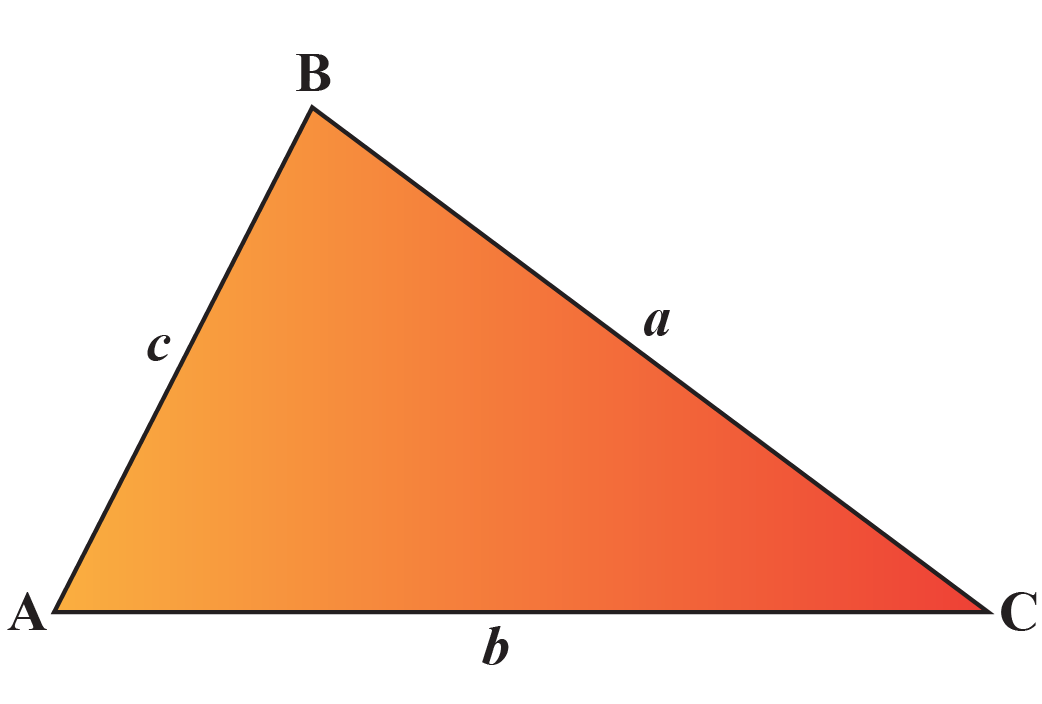Then, the law of cosine states that:

 $a^{2}=b^{2}+c^{2}-2bc\cos{A}$

The law of cosine is also known as the cosine rule.

This law is useful to find the missing information in any triangle.

For example, if you know the lengths of two sides of a triangle and an angle included between them, this rule helps to find the third side of the triangle.

## What Are General Formulas For Law of Cosine?

### Statement

The law of cosine states that the square of any one side of a triangle is equal to the difference between the sum of squares of the other two sides and double the product of other sides and cosine angle included between them.

### Examples of General Formulas

There are three versions of the cosine rule.

 $a^{2}=b^{2}+c^{2}-2bc\cos{A}$
 $b^{2}=a^{2}+c^{2}-2ac\cos{B}$
 $c^{2}=a^{2}+b^{2}-2ab\cos{C}$

If you plug $$90^{\circ}$$ for the angle in one of the rules, what will happen?

Since $$\cos{90^{\circ}}=0$$, we are left with the generalization of the Pythagoras theorem.

## How to Prove the Law of Cosine?

### Law of Cosine: Proof

There is more than one way to prove the law of cosine.

Let's prove it using trigonometry.

Consider the following figure.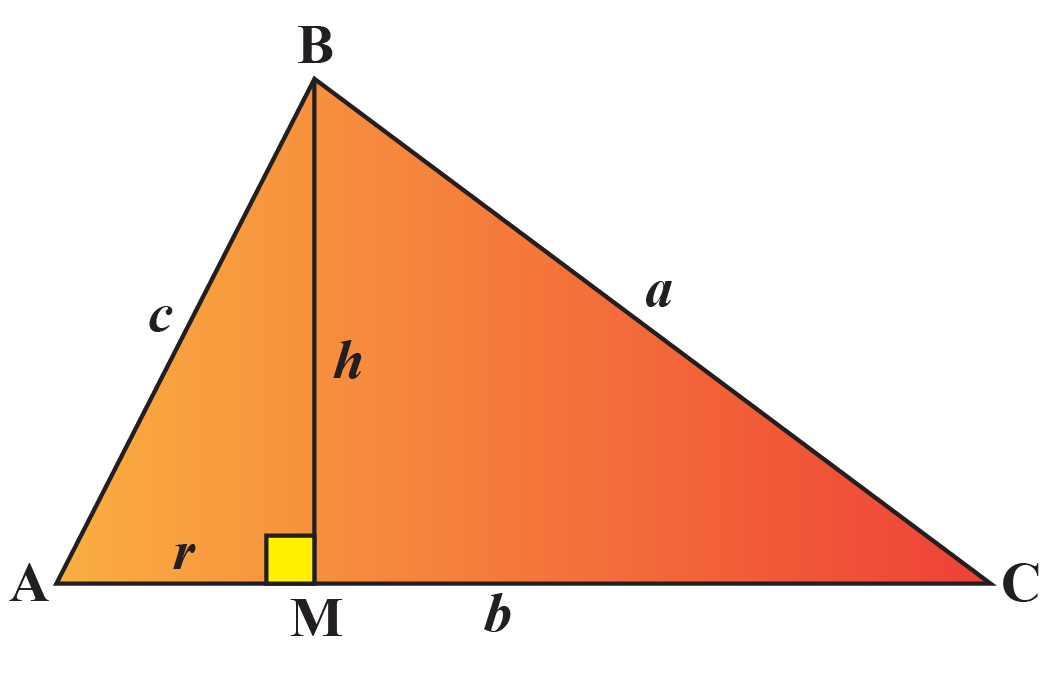In $$\Delta ABM$$ we have,

\begin{align}\sin{A}=\dfrac{BM}{AB}=\dfrac{h}{c}\;\;\;\;\;\; \cdots (1)\end{align}

and,

\begin{align}\cos{A}=\dfrac{AM}{AB}=\dfrac{r}{c}\;\;\;\;\;\; \cdots (2)\end{align}

From equation (1) and (2), we get $$h=c(\sin{A})$$ and $$r=c(\cos{A})$$

By Pythagorus Theorem in $$\Delta BMC$$,

\begin{align}a^{2}=h^{2}+(b-r)^{2}\;\;\;\;\;\; \cdots (3)\end{align}

Substitute $$h=c(\sin{A})$$ and $$r=c(\cos{A})$$ in equation (3).

\begin{align}a^{2}&=(c(\sin{A}))^{2}+(b-c(\cos{A}))^{2}\\&=c^{2} \sin^{2}{A}+b^{2}+c^{2} \cos^{2}{A}-2bc\cos{A}\\&=c^{2} (\sin^{2}{A}+\cos^{2}{A})+b^{2}-2bc\cos{A}\\&=c^{2}+b^{2}-2bc\cos{A}\end{align}

Example

Experiment with the simulation below to visualize the proof of law of cosine.

How to Find Missing Length and Angles using the Law of Cosine?

Let us consider a few examples to find the missing side and angle of a triangle.

Example 1

Look at the figure shown below.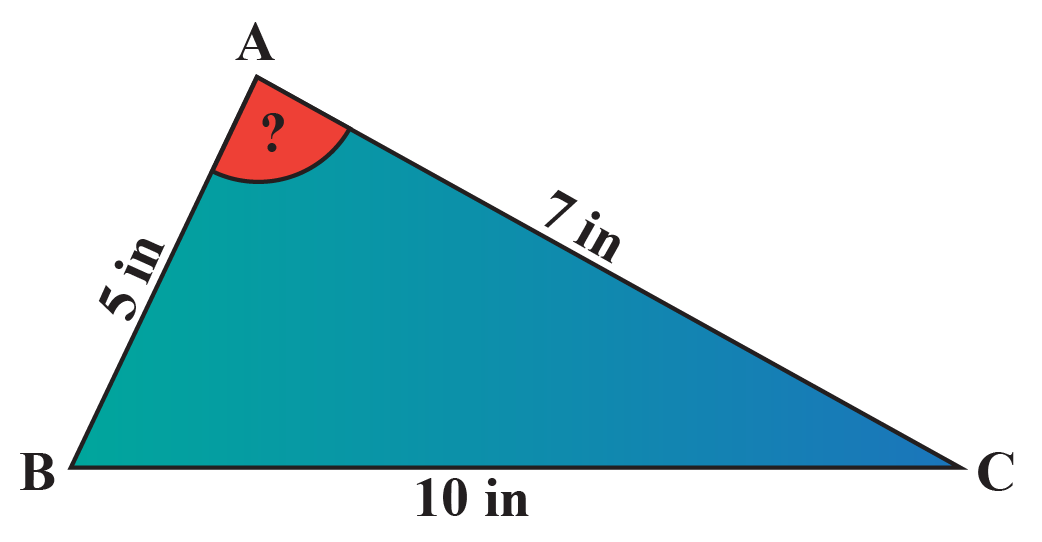We need to find the measure of $$\angle A$$.

We will use the formula $$a^{2}=b^{2}+c^{2}-2bc\cos{A}$$.

Substitute 10 for $$a$$, 7 for $$b$$ and 5 for $$c$$.

\begin{align}a^{2}&=b^{2}+c^{2}-2bc\cos{A}\\10^{2}&=(7)^{2}+(5)^{2}-2(5)(7)\cos{A}\\70\cos{A}&=-26\\\cos{A}&=-\dfrac{13}{35}\\A&=111.8^{\circ}\end{align}

In this example, we used the law of cosine equation to find the missing angle.

Now, let us use the law of cosine equation to find the missing side.

Example 2

Look at the figure below and let us find the missing side.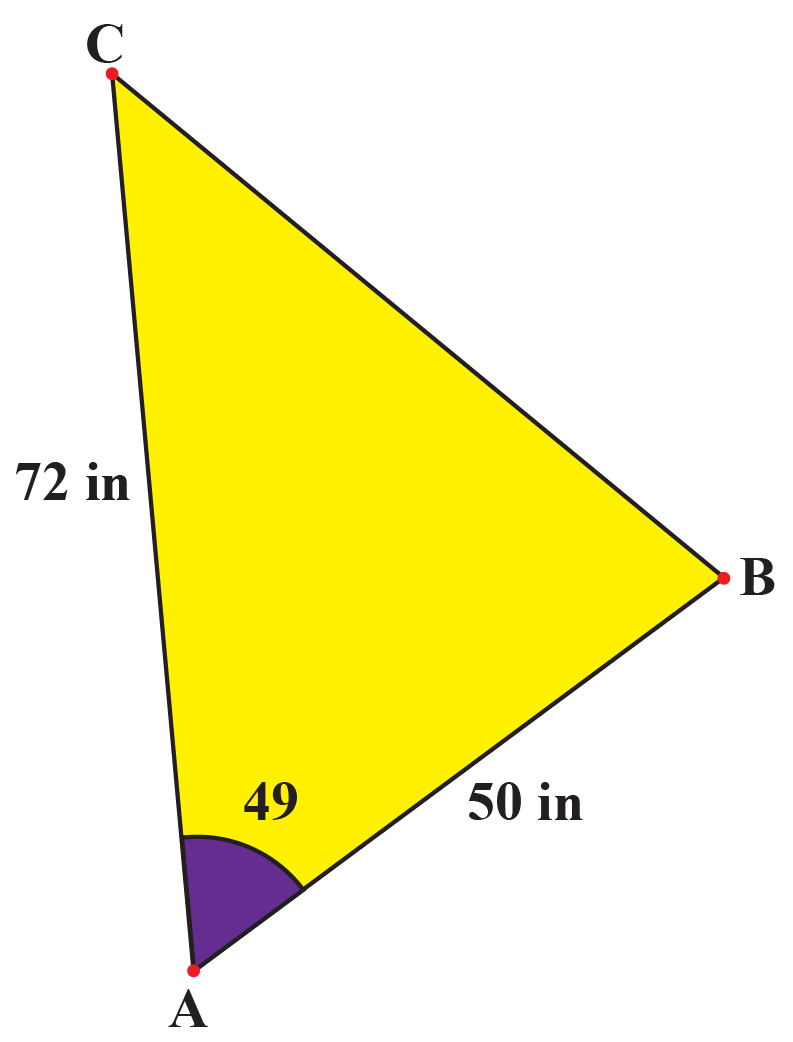Substitute 72 for $$b$$, 50 for $$c$$ and $$49^{\circ}$$ for $$A$$.

\begin{align}a^{2}&=b^{2}+c^{2}-2bc\cos{A}\\a^{2}&=(72)^{2}+(50)^{2}-2(72)(50)\cos{49^{\circ}}\\a^{2}&=5184+2500-(7200)(0.656)\\a^{2}&=5184+2500-4723.2\\a^{2}&=2960.8\\a&\approx 54.4\end{align}

So, the missing length of the side is 54.4 inches.

## Solved Examples

 Example 1

Neil wants to determine the distance between the two boats floating at the lake.

The figure given below shows that the boy is standing at point $$A$$ and two boats are located at points, $$B$$ and $$C$$.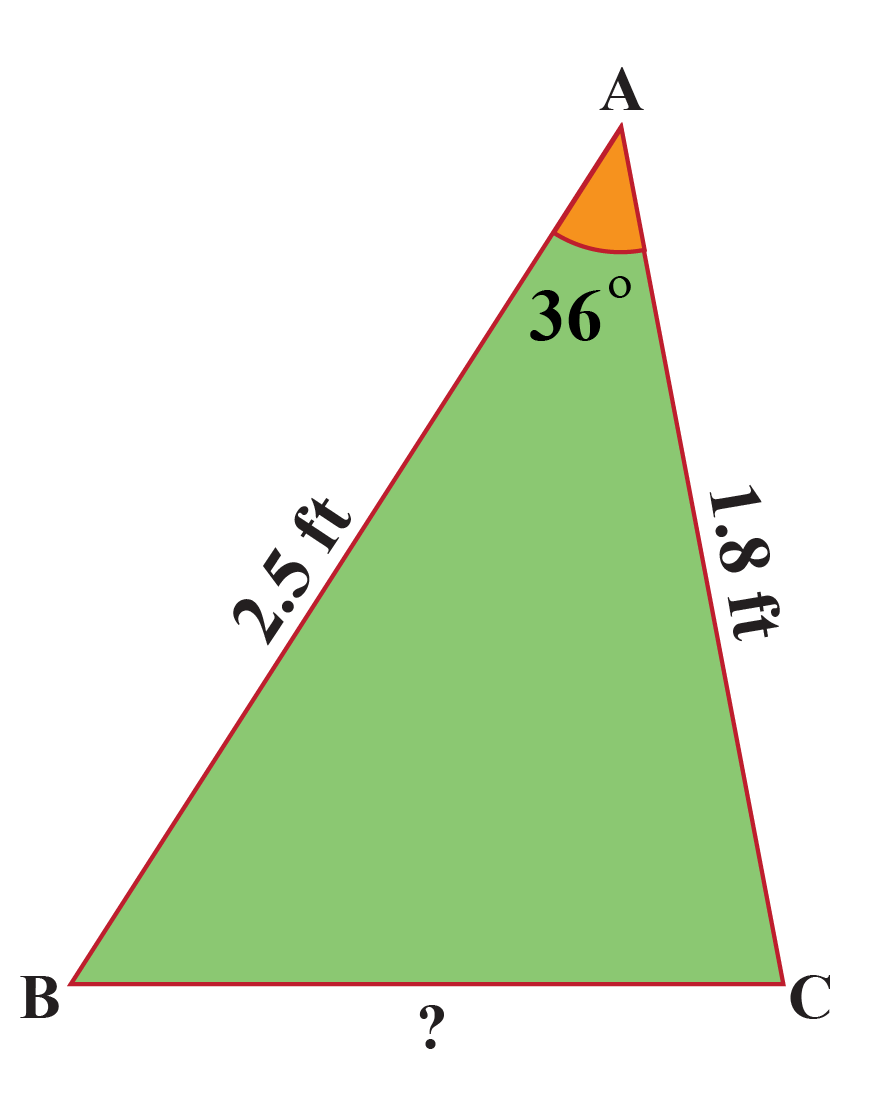Can you help him calculate the required distance?

### Solution

The law of cosine is expressed in three different ways.

We will use the formula $$a^{2}=b^{2}+c^{2}-2bc\cos{A}$$, because the required side is opposite to $$\angle A$$ and the given sides form $$\angle A$$.

Substitute 1.8 for $$b$$, 2.5 for $$c$$ and $$36^{\circ}$$ for angle $$A$$.

\begin{align}a^{2}&=b^{2}+c^{2}-2bc\cos{A}\\a^{2}&=(1.8)^{2}+(2.5)^{2}-2(2.5)(1.8)\cos{36^{\circ}}\\a^{2}&=3.24+6.25-(9)(0.8)\\a^{2}&=3.24+6.25-7.2\\a^{2}&=2.29\\a&\approx 1.5\end{align}

 So, the length across the boats is $$1.5\;\text{feet}$$.
 Example 2

A farmer has a huge field in the shape of a triangle.

He wants to do fencing around his field.

He knows the dimensions of his field as shown in the figure below.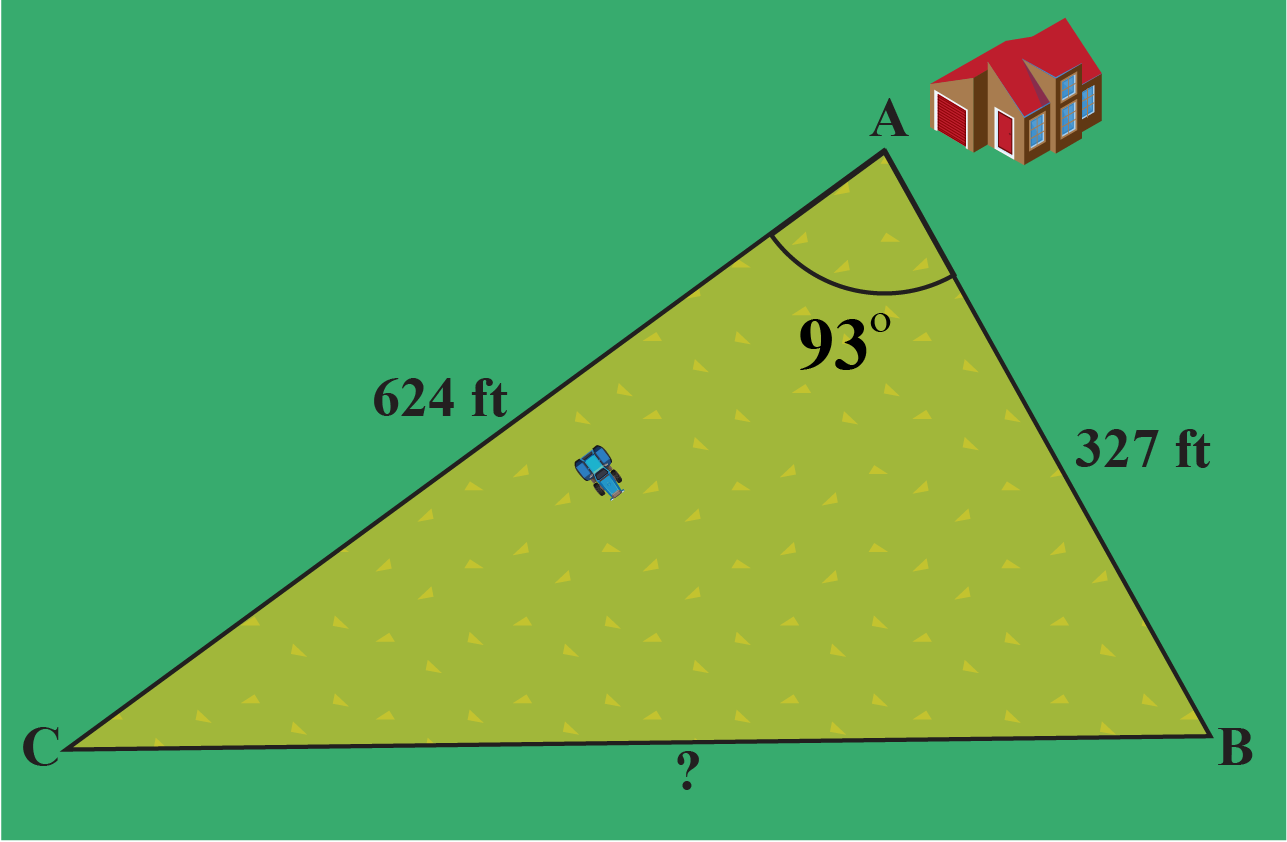Can you calculate how much fencing is needed to enclose the field?

Solution

We will use the definition of law of cosine: $$a^{2}=b^{2}+c^{2}-2bc\cos{A}$$.

Substitute 624 for $$b$$, 327 for $$c$$ and $$93^{\circ}$$ for $$A$$.

\begin{align}a^{2}&=b^{2}+c^{2}-2bc\cos{A}\\a^{2}&=(624)^{2}+(327)^{2}-2(624)(327)\cos{93^{\circ}}\\a^{2}&=389376+106929-(408096)(-0.05)\\a^{2}&=389376+106929+20404.8\\a^{2}&=516709.8\\a&\approx 719\end{align}

So, the perimeter of the triangular field is $$624\;\text{ft.}+327\;\text{ft.}+719\;\text{ft.}=1670\;\text{ft.}$$

 So, the farmer will need $$1670\;\text{ft.}$$ of fencing.
 Example 3

Look at the parallelogram shown below.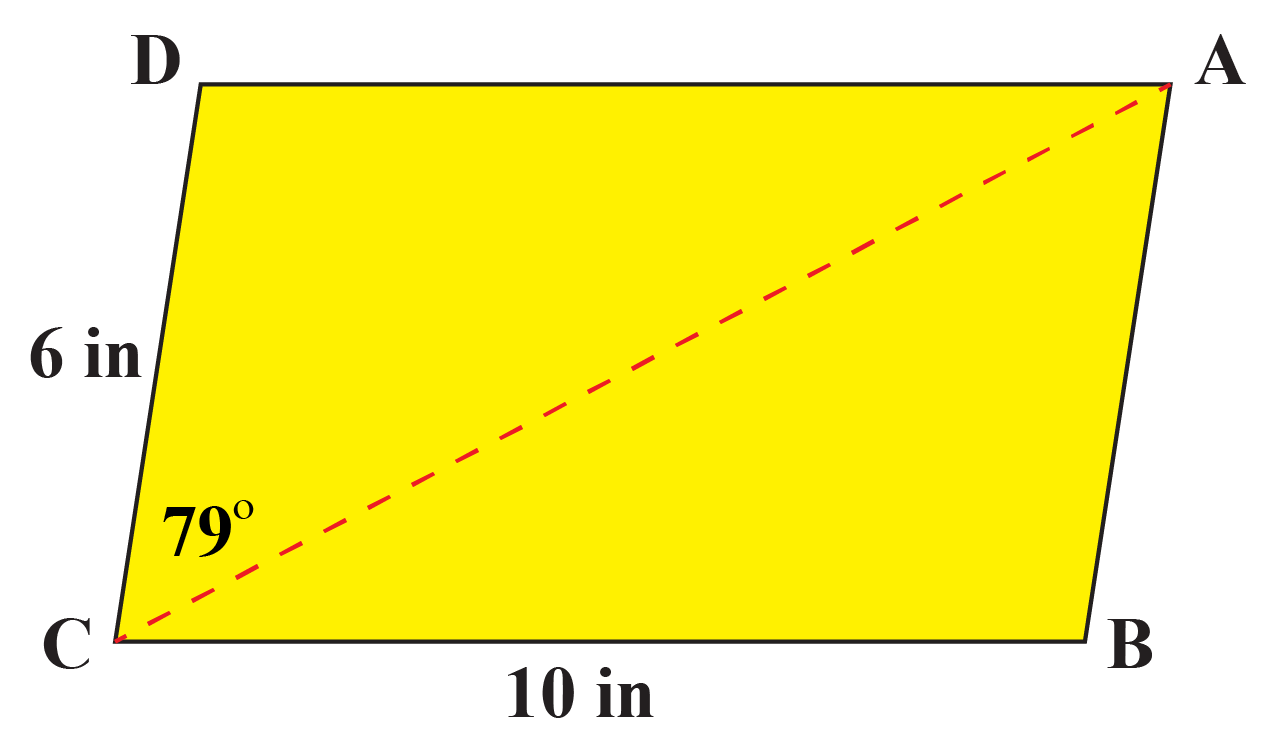You are given two of its side as 6 inches and 10 inches and an angle including them is of measure $$79^{\circ}$$.

Can you determine the length of the diagonal of the parallelogram?

Solution

We know that the adjacent angles of a parallelogram are supplementary.

So, $$\angle B = 180^{\circ} - \angle C=180^{\circ} - 79^{\circ} =101^{\circ}$$.

Also, the opposite sides of a parallelogram are equal.

So, $$AB=CD=6\;\text{in.}$$.

Let's apply the cosine rule in $$\Delta ABC$$.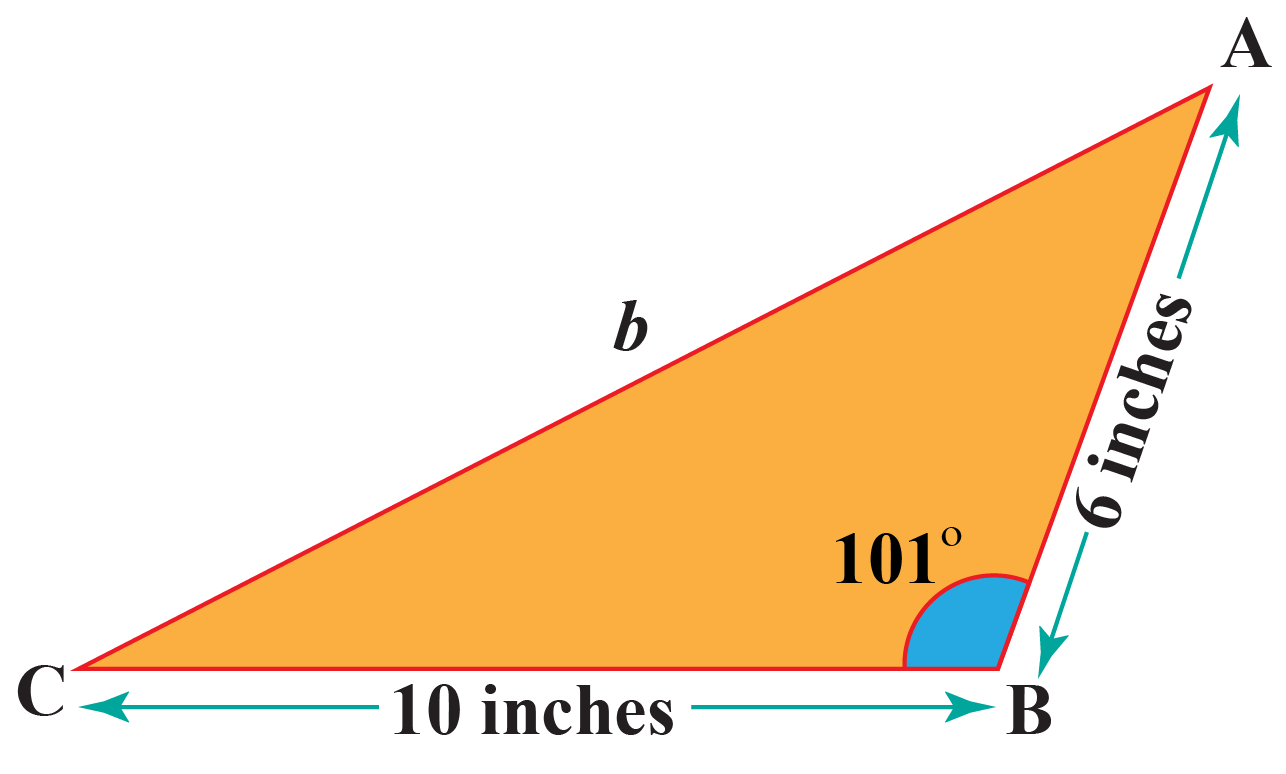Substitute 10 for $$a$$, 6 for $$c$$ and $$101^{\circ}$$ for $$B$$.

\begin{align}b^{2}&=a^{2}+c^{2}-2ac\cos{B}\\b^{2}&=(10)^{2}+(6)^{2}-2(10)(6)\cos{101^{\circ}}\\b^{2}&=100+36-(120)(-0.19)\\b^{2}&=100+36+22.8\\b^{2}&=158.8\\b&\approx 12.6\end{align}

 So, the length of the diagonal is approximately 12.6 inches.Challenging Questions
 1. A spider is lost in its web. Look at the figure below.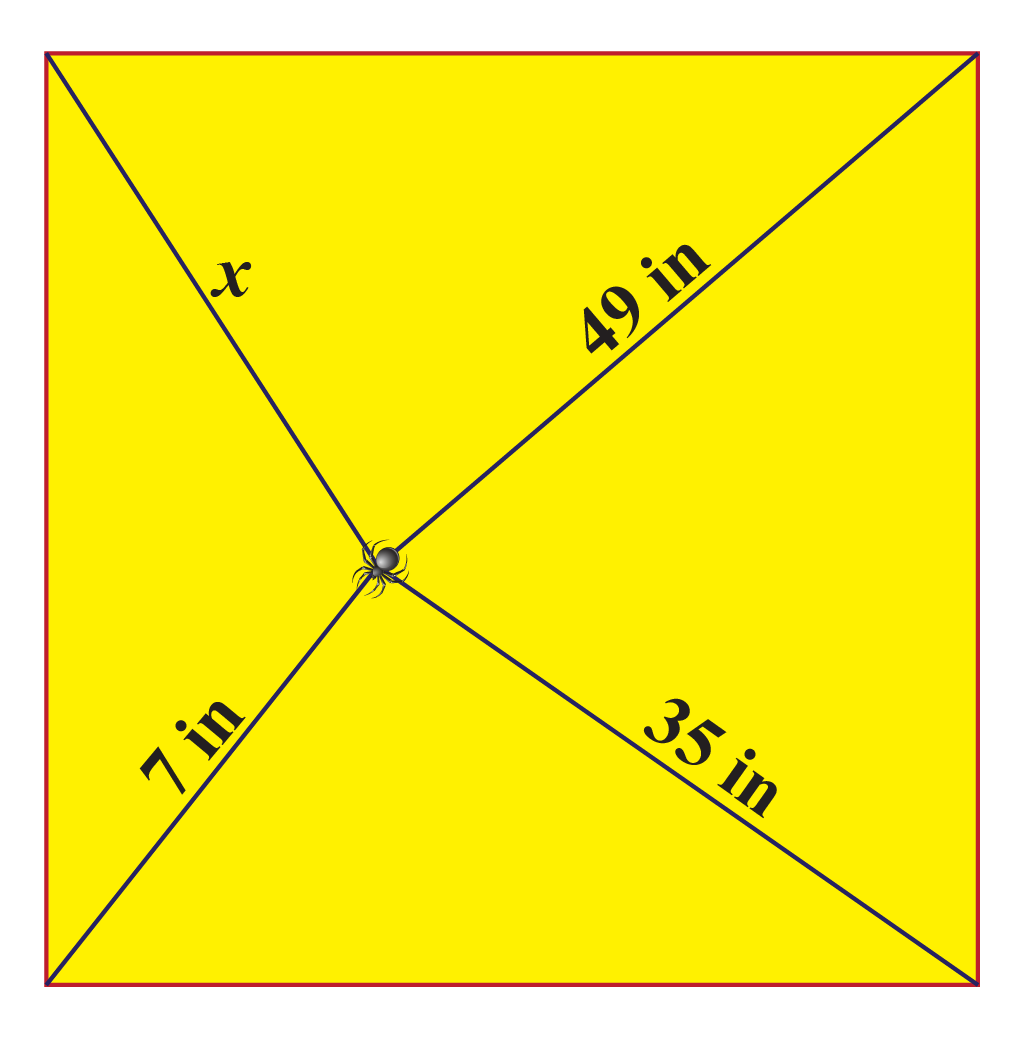Can you find the value of $$x$$?

## Interactive Questions

Here are a few activities for you to practice.

## Let's Summarize

This mini-lesson targeted the fascinating concept of the Law of Cosine. The math journey around the Law of Cosine started with what a student already knew and went on to creatively crafting a fresh concept in the young minds. Done in a way that is not only relatable and easy to grasp, but will also stay with them forever.

At Cuemath, our team of math experts is dedicated to making learning fun for our favorite readers, the students!

Through an interactive and engaging learning-teaching-learning approach, the teachers explore all angles of a topic.

Be it worksheets, online classes, doubt sessions, or any other form of relation, it’s the logical thinking and smart learning approach that we, at Cuemath, believe in.

## 1. Does cosine law work for all triangles?

Yes, the law of cosine can be applied in any triangle.

## 2. How do you derive the law of cosine?

There is more than one way to derive the law of cosine.

A few of them are given below.\:

• Using basic concepts of trigonometry.
• Using vector algebra.
• Using the law of sine.
• Using the coordinate geometry to find the distance between two coordinate points.

## 3. What is the law of sine?

Look at the triangle shown below: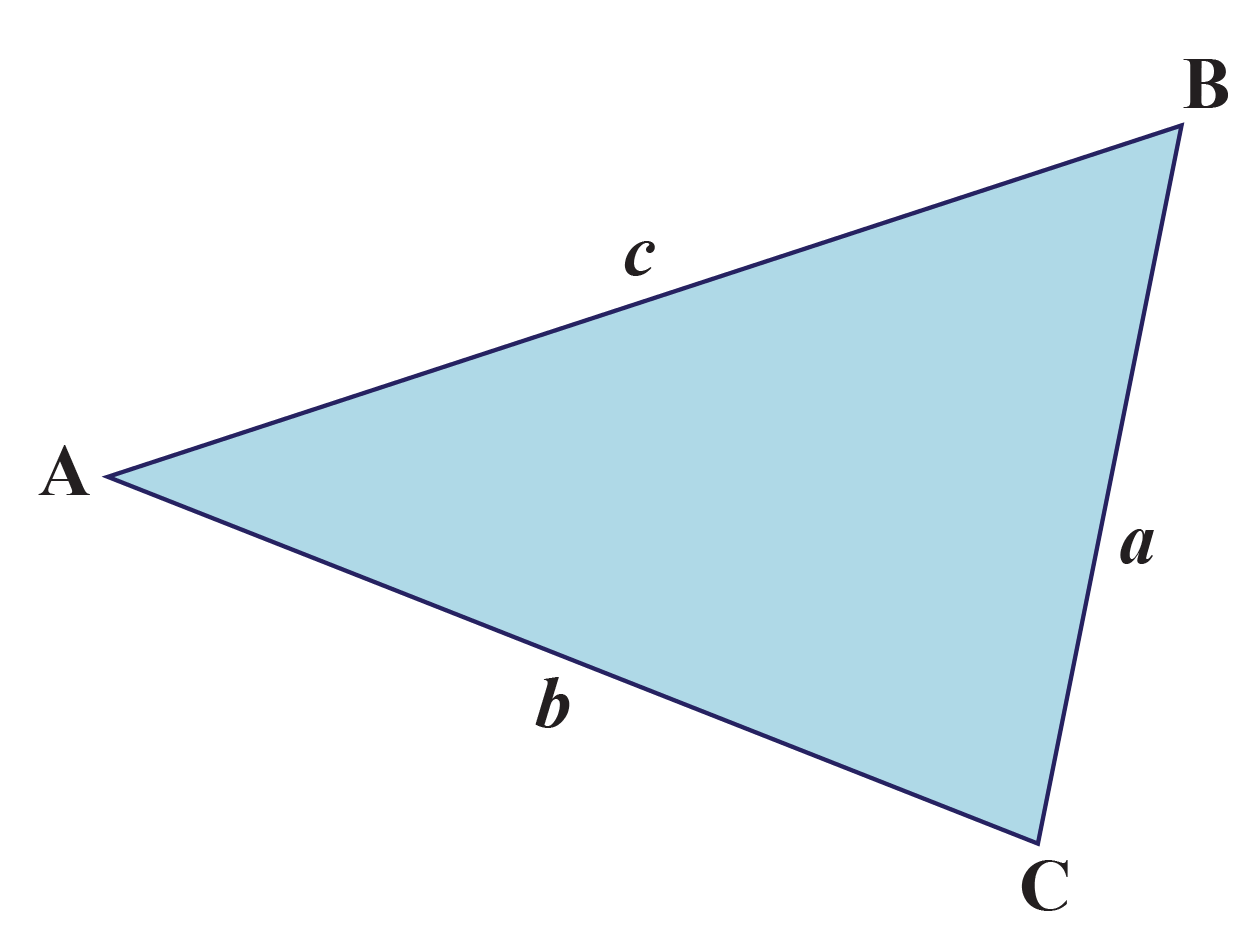The law of sine states that $$\dfrac{\sin{A}}{a}=\dfrac{\sin{B}}{b}=\dfrac{\sin{C}}{c}$$.

More Important Topics
Numbers
Algebra
Geometry
Measurement
Money
Data
Trigonometry
Calculus